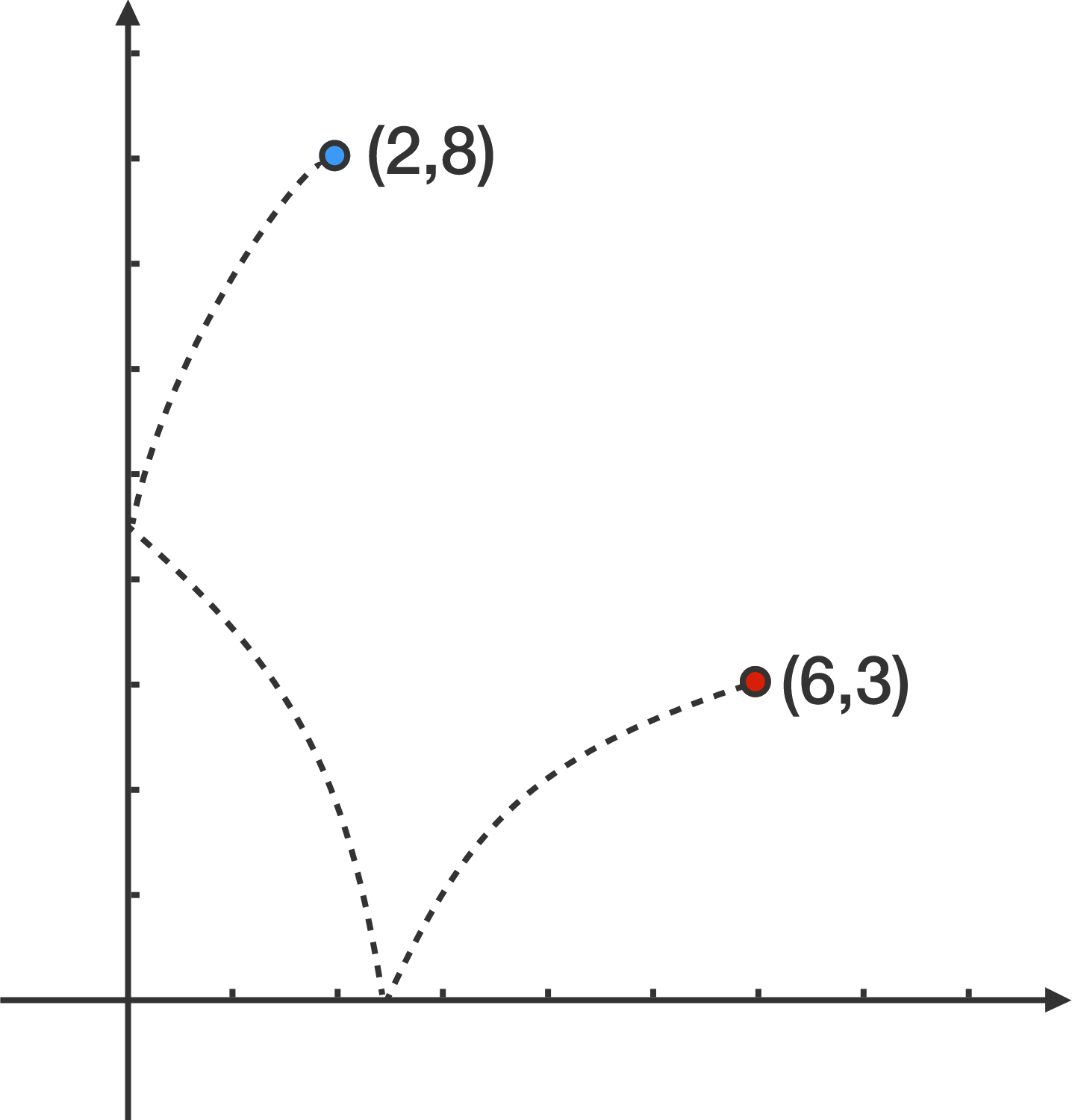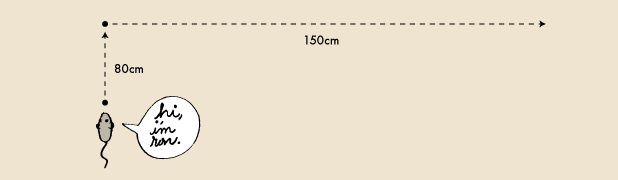Geometry

# 2D Coordinate Geometry: Level 2 Challenges

A man walks 5 meters north from his house, turns left $90^\circ$, walks 12 meters further and reaches a park. How far, in meters, is the park from his house?

Three of the vertices of a square have coordinates of $(9, 6), ( 12, 10)$, and $(5, 9 )$. What are the coordinates of the last vertex?

You are given four points $(1, 3), (-3, 5), (-5, -3),$ and $(7, -1).$ Suppose two line segments are to be constructed so that each point is used exactly once. What is the greatest possible distance between the midpoints of two such line segments?Find the length of the shortest path that can be drawn from the point $(6, 3)$ to the point $(2, 8)$ such that the path touches the $x$-axis and the $y$-axis once.Ron the Mouse travels 80 centimeters north and then 150 centimeters east.

Let $d$ be the distance traveled by Ron the Mouse and $s$ be the magnitude of the displacement of the mouse.

Find the value (in centimeters) of $d-s.$

×